# Inductor for Power LinesPower Inductor Basic Course - Chapter 3-1

## Chapter 3: What are important DC-DC converter characteristics?

In Chapter 2, we talked about the types of DC-DC converters and their operation mechanisms. The key power inductor parameters, however, cannot be determined from this information alone. In order to understand how converter characteristics work with these parameters, we first need to look into the important DC-DC converter characteristics required by the power inductors.

### 3.1 Important DC-DC converter characteristics

There are many different characteristics that are required of DC-DC converters. Of these characteristics, the following three affect the performance of power inductors the most:

①Efficiency, ②ripple voltage, and ③load response

Read on to learn more about these characteristics and what they have to do with the power inductor.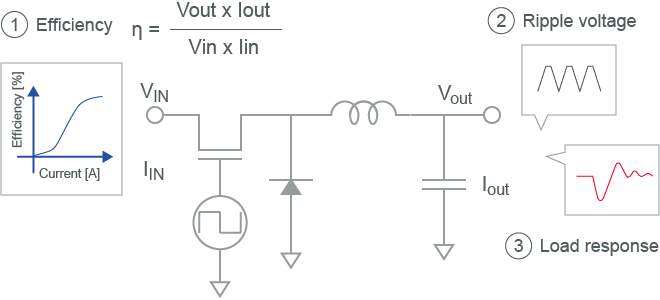Figure 3-1 Important DC-DC converter characteristics

### 3.2 Efficiency

First, let’s talk about power efficiency. In the ideal DC-DC converter where there is no power loss, the input voltage is equivalent to the output voltage. If this is achieved, efficiency is at 100%. However, for an actual DC-DC converter, there is power loss equivalent to PDC-DC, making the output voltage lower than the input voltage. Efficiency is demonstrated in the following equation. The lower the PDC-DC value, the better the efficiency of the converter.
The efficiency mentioned in this chapter refers not only to the efficiency of the power inductor but also to the efficiency of the DC-DC converter as a whole.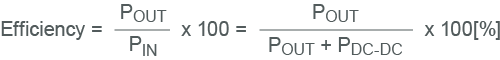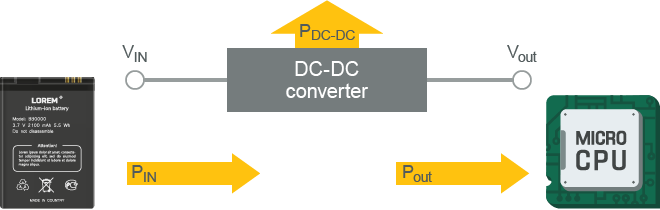Figure 3-2-1 DC-DC converter power consumption

Figure 3-2-2 gives an example of a measurement of efficiency. Because the current supplied to the load (Iout) changes depending on the application of the converter, this is often used as the X axis value.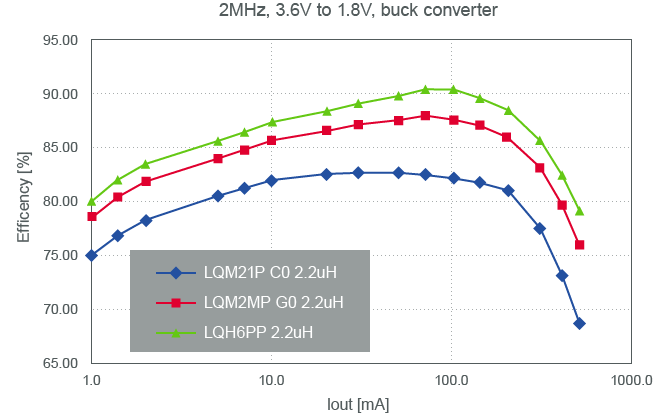Figure 3-2-2 Example of DC-DC converter efficiency

PDC-DCpower consumed by the DC-DC converter can be mainly broken down into PIC, PPI、Pother, and Pother power. PIC is power loss that occurs in the IC, and includes the losses that occur from switching and on-resistance. PPI is the loss that occurs from the inductor. Pother is the loss that occurs from the capacitor ESR as well as other instances of power loss.
While it also depends on the operational requirements and IC performance, PPI can make up about 50% of the PDC-DC power consumed in a converter. This affects the efficiency of the power inductor greatly, which creates a demand for power inductors to have low power loss as a characteristic.Figure 3-2-3 Power Load resistance Breakdown of power consumption

The inductor power loss PPI can be broken down into DC loss and AC loss, as demonstrated in the equation below. DC loss shown here is power loss from a direct current while AC loss is power loss from an alternating current. DC loss is proportional to Rdc (direct current resistance) because it is the conductor power loss from a direct current passing through a coil of wire. Meanwhile, AC loss is proportional to Rac (alternating current resistance) and is defined as the power loss in the conductor from an alternating current passing through a coil of wire. AC loss includes power loss in the core, which is also referred to as iron loss. At high frequency, power loss in the conductor also tends to increase due to the skin effect. Rac here is defined in the following equation. Rac1 is the conductor resistance that increases due to the skin effect while Rac2 is the resistance of the core material.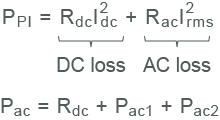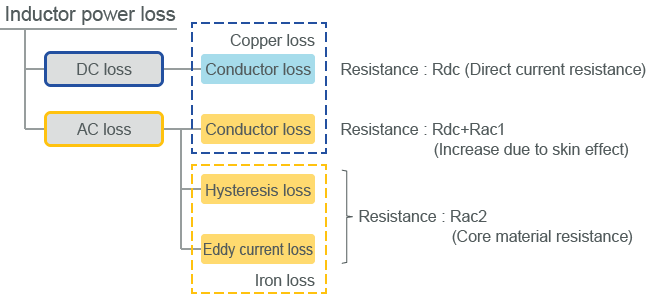Figure 3-2-4 Breakdown of inductor power loss

Figure 3-2-5 is a depiction of AC loss and DC loss in relation to the load current. The AC components in the current supplied to the inductor are determined by the input/output voltage and frequency. Because of this, the amount of AC loss is not altered greatly by changes to the load current. DC loss, on the other hand, is proportional to the square of the load current. The DC loss is low due to the small current at low load, but it increases greatly when the load current increases. Therefore, AC loss is dominant in low load density areas, and DC loss is dominant in high load density areas.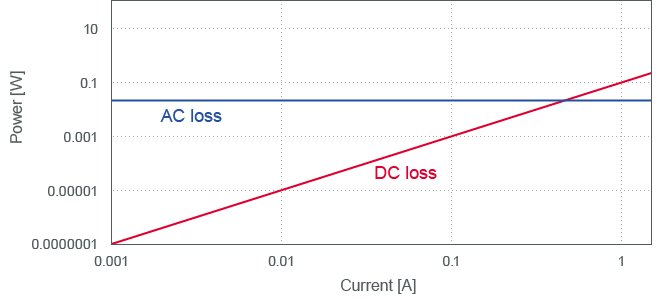Figure 3-2-5 Power loss through low load or high load dominance

DC-DC converter Inductance Efficiency Simulator Operation frequency Input voltage Output voltage Output current Efficiency evaluation through simulation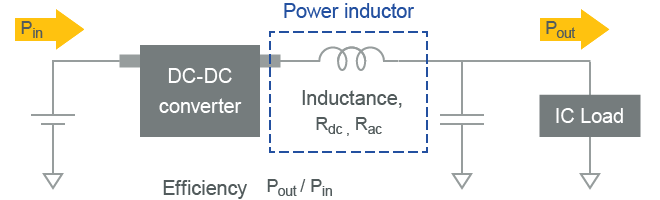• Simulator : LTSPICE
• DC-DC converter : Linear Tech LTC3612
• Operation frequency : 4MHz
• Input voltage : 3.6V
• Output voltage : 1.8V
• Output current Iout : 10m～3A

Figure 3-2-6 Efficiency evaluation through simulation

Figure 3-2-7 shows the resulting efficiency when Rdc is between 0.01Ω and 2.0Ω. Because Idc is small at low load, there is not a big change to efficiency even when Rdc changes. However, because Idc is large at high load, a change in Rdc has a large impact on efficiency.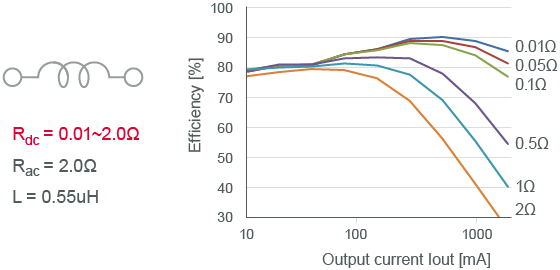Figure 3-2-7 How Rdc affects efficiency

The resulting efficiency from changing Rac from 0.1Ω to 10Ω is shown in the following. Because the amount of Rac accounts for the amount of AC loss, low load, which is when AC loss is dominant, greatly impacts efficiency. However, because DC loss is dominant due to the increase in Idc at high load, there is not a big change to efficiency even when there is a change to Rac.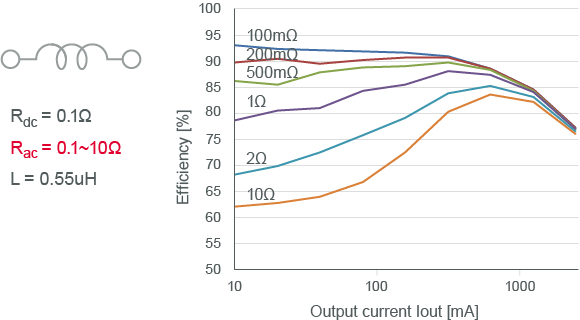Figure 3-2-8 How Rac affects efficiency

Finally, we have the resulting efficiency when the inductance is between 0.22μH and 2μH. Like Rac, inductance greatly impacts AC loss. Therefore, there is a great impact on efficiency at low load and little change to efficiency at high load.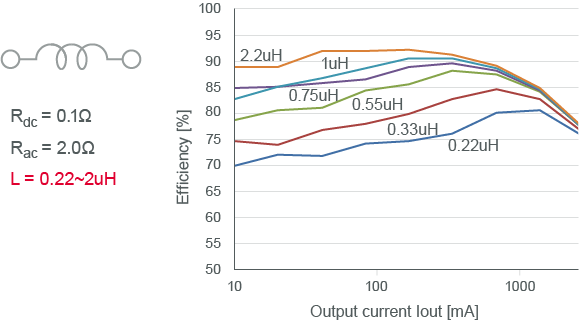Figure 3-2-9 How inductance affects efficiency

Inductance impacts efficiency because the AC components in the current supplied to the inductor are determined by the inductance. The slope of the triangular wave current is proportional to the inverse of the inductance. Therefore, if the inductance is high, the current amplitude decreases, and AC loss is reduced.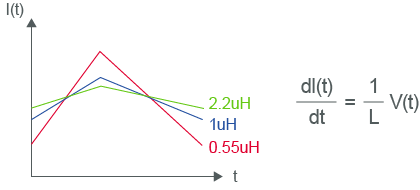Figure 3-2-10 Why inductance impacts efficiency

Considering what was mentioned up until this point, important characteristics for reducing inductor power loss are low Rac and high inductance at low load, and low Rdc at high load.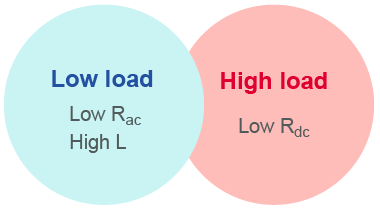Figure 3-2-11 Inductor characteristics required for high efficiency